V. INVERSE TRIGONOMETRIC FUNCTIONS

1. (i) Sketch the graph of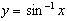. State the domain and range. Does y has a minimum value ? If so, is this a stationary value on the curve ?

(ii) For what values of x is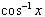defined ? Sketch the graph of y =, stating its range.

(iii) Define the function y =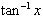, and sketch it. Does y has a maximum value ? Explain your answer from the sketch.

2. Without using calculator, find the value of:

(a)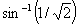(b)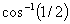(c)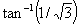(d)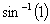(e)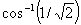(f)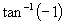(g)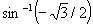(h)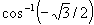(i)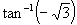3. Find the exact value of

(a)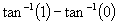(b)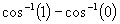(c)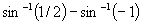(d)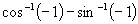4. Evaluate without tables:

(a)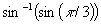(b)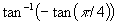(c)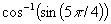(d)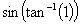(e)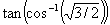(f)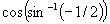5. Without tables, find the value of:

(a)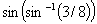(b)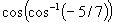(c)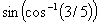(d)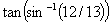(e)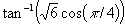(f)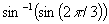6. (i) Write down the result of sin(A + B) and hence deduce the result for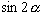. Use this to evaluate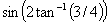.

(ii) Write down a result for cos(x + y) and show that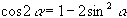. Hence deduce the exact value of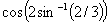7. Determine the domain and range of each of the following functions:

(a)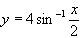(b)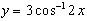(c)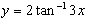8. State the domain and range each of the following functions:

(a)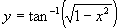(b)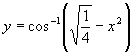(c)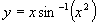9. (i) Find the maximum value of the function 2x(1 - x) and hence determine the range of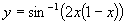for 0 < x < 1.

(ii) Find the least value of x(x - 2) and hence determine the range of :

(a)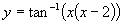(b)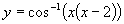if for 0 < x < 2.

10. State the domain and range of cosx . Hence determine the domain and range of each of the following functions:

(a)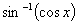(b)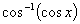(c)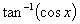(d)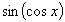(e)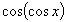(f)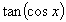11. If n is a positive integer, determine the limits of the following sequences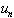when:

(a)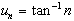(b)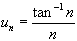(c)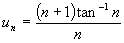(d)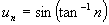(e)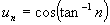(f)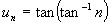12. Determine whether each of the following functions is an even or odd:

(a) y = sinx (b)(c)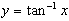(d)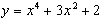(e)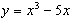(f)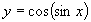(g)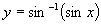(h)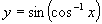(i)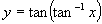13. (i) Prove that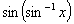is an odd function.

(ii) Provided -1 < x < 1 show that= x .

(iii) Hence, draw the graph of y =.

(iv) Using a similar method to show that

(a)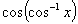for -1 < x < 1 (b)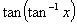for all x.